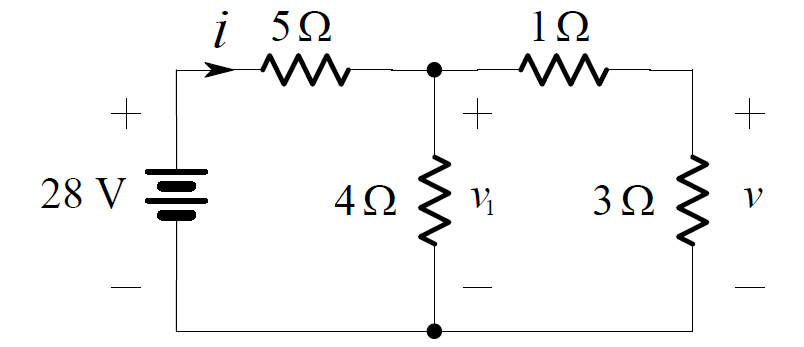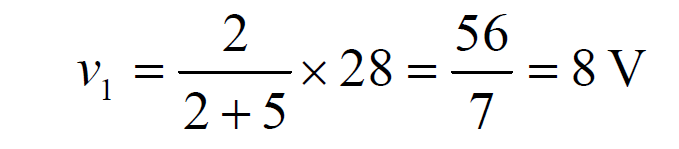# Voltage Divider Rule

It can be quite useful to determine how a voltage appearing across two series resistors “divides” between them.

Consider the circuit shown below:By Ohm’s Law, the current in the resistors is:By application of Ohm’s Law again, the voltage across R1  is:

v1 = R1 . i

and therefore:Similarly, the voltage across R2  is:These equations describe how the voltage is divided between the resistors. Because of this, a pair of resistors in series is often called a voltage divider.

Example:

We want to find the voltage v in the circuit below:Combining the series connection of the 1 ohm and 3 ohm resistors, we obtain theNow the pair of 4 ohms resistors in parallel can be combined as shown below:By voltage division:Returning to the original circuit and applying voltage division again yields: